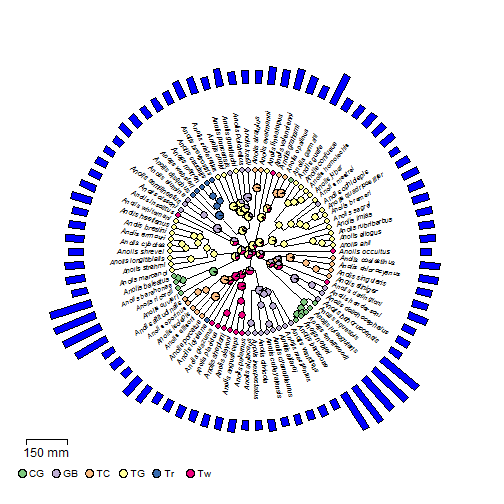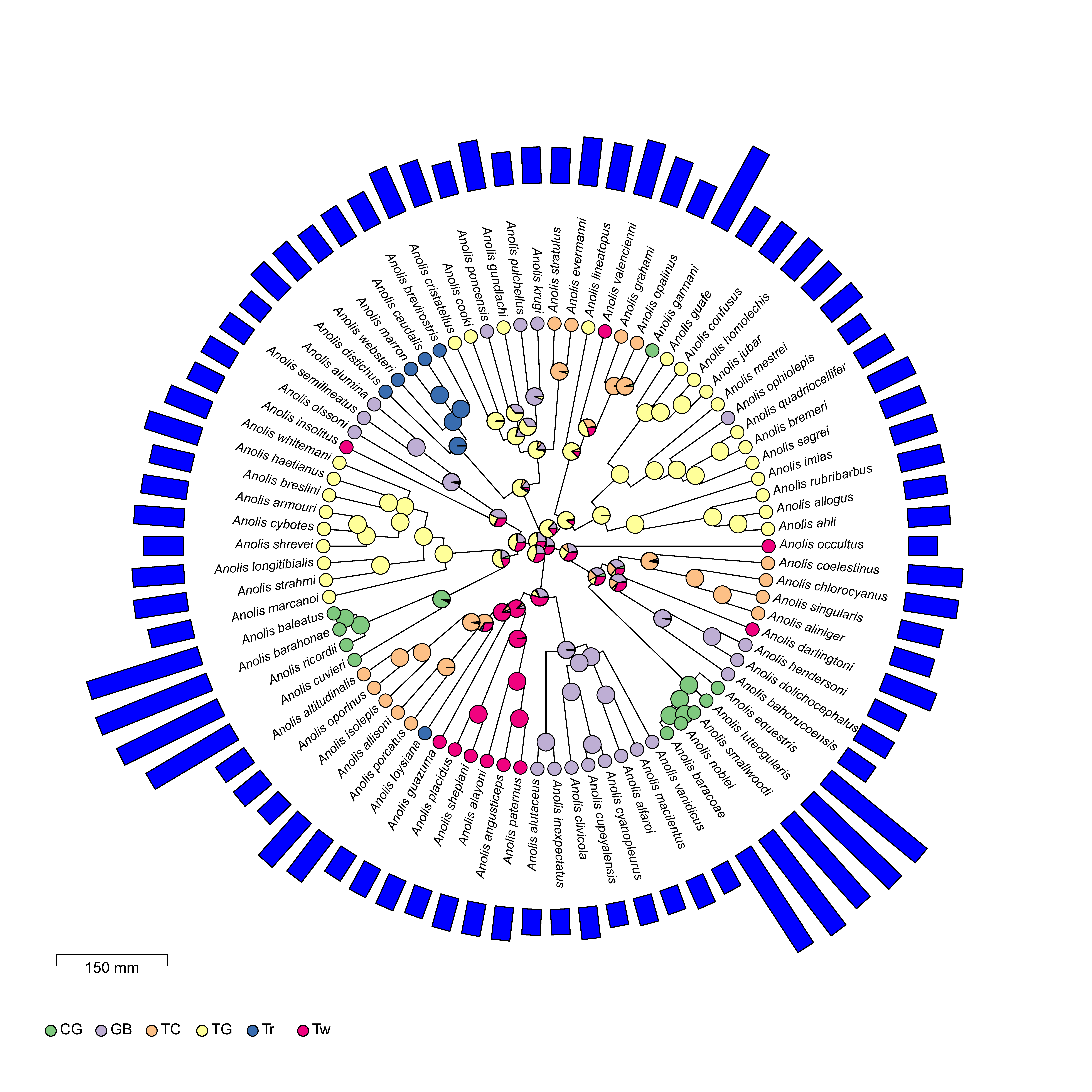## Thursday, November 16, 2017

### Even 'hackier' solution to offsetting the tip labels in a plotted fan-style tree

In the not too distant past I posted a 'hack' to offset the tip labels of a fan-style tree plotted with phytools.

Unfortunately, this hack won't work for some functions that use `plotTree(...,type="fan")` internally, such as `plotTree.wBars`. This becomes important when one, say, wants to include (say) node labels on a plotted tree with bars.

Here's an even 'hackier' hack that does. I'm going to use a tree of Anolis lizards along with some real data for SVL (body size).

Here goes:

``````## first load libraries
library(phytools)
## this will be our tree
data(anoletree)
## I'm going to use this later
ecomorph<-as.factor(getStates(anoletree,"tips"))
## convert my "simmap" object to a regular old tree
tree<-as.phylo(anoletree)
## plus make sure the tips are in 'cladewise' order
## get our data
svl<-setNames(X[,"SVL"],rownames(X))
## fix the names
names(svl)<-paste("Anolis",names(svl),sep="_")
## sort into the order of the tip labels & back transform to original scale
## (for fun)
svl<-exp(svl[tree\$tip.label])
## now identify the tip labels on each side of our fan!
ii<-c(1:floor(0.25*Ntip(tree)),
ceiling(0.75*Ntip(tree)):Ntip(tree))
jj<-setdiff(1:Ntip(tree),ii)
hack<-tree
hack\$tip.label[ii]<-paste("  ",tree\$tip.label[ii],sep="")
hack\$tip.label[jj]<-paste(tree\$tip.label[jj],"  ",sep="")
SCALE<-0.02 ## for later
plotTree.wBars(hack,setNames(svl,hack\$tip.label),
type="fan",tip.label=T,fsize=0.6,scale=SCALE,col="blue",
width=0.5,lwd=1)
## now our labels!
mtrees<-make.simmap(tree,ecomorph,model="ER",nsim=100)
``````
``````## make.simmap is sampling character histories conditioned on the transition matrix
##
## Q =
##             CG          GB          TC          TG          Tr          Tw
## CG -0.11570723  0.02314145  0.02314145  0.02314145  0.02314145  0.02314145
## GB  0.02314145 -0.11570723  0.02314145  0.02314145  0.02314145  0.02314145
## TC  0.02314145  0.02314145 -0.11570723  0.02314145  0.02314145  0.02314145
## TG  0.02314145  0.02314145  0.02314145 -0.11570723  0.02314145  0.02314145
## Tr  0.02314145  0.02314145  0.02314145  0.02314145 -0.11570723  0.02314145
## Tw  0.02314145  0.02314145  0.02314145  0.02314145  0.02314145 -0.11570723
## (estimated using likelihood);
## and (mean) root node prior probabilities
## pi =
##        CG        GB        TC        TG        Tr        Tw
## 0.1666667 0.1666667 0.1666667 0.1666667 0.1666667 0.1666667
``````
``````## Done.
``````
``````obj<-summary(mtrees)
library(RColorBrewer)
cols<-setNames(brewer.pal(length(levels(ecomorph)),"Accent"),
levels(ecomorph))
nodelabels(pie=obj\$ace,piecol=cols,cex=0.4)
tiplabels(pie=to.matrix(ecomorph,levels(ecomorph)),
piecol=cols,cex=0.3)
## simmap legend?
x<-0.9*par()\$usr
y<-0.9*par()\$usr
x=x,y=y,shape="circle",fsize=0.8)
y<-0.75*par()\$usr
lines(x=x+c(0,150*SCALE),y=rep(y,2))
lines(x=x+rep(0,2),y-c(0,0.01*diff(par()\$usr[3:4])))
lines(x=x+rep(150*SCALE,2),y-c(0,0.01*diff(par()\$usr[3:4])))
text(mean(x+c(0,150*SCALE)),y,"150 mm",pos=1)
``````Here's a higher quality version:(Click to enlarge.)

You can do anything in R!